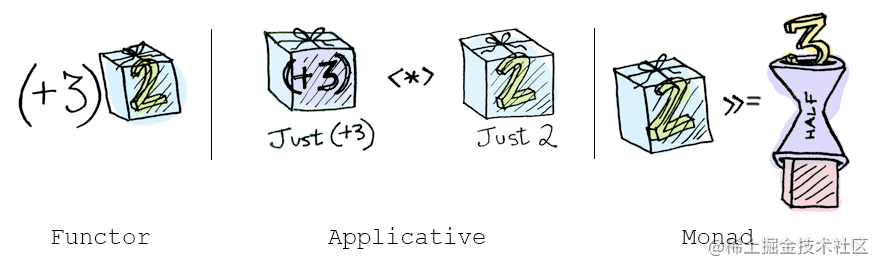网易云音乐

• 如何解决嵌套的 `try-catch`
• 异步函数的组合
• Promise 也是一种 Functor ?

## 嵌套的 Array

Javascript Array 的 `map` 方法，相信开发者们都很熟悉，并且几乎在每天的编程中都会用到，但 Array 原型链上的另一个方法 `Array.prototype.flatMap` 可能很多人没怎么用过，从字面意思上理解就是`扁平化的 map`，实际作用也确实是的，看一个和 `map` 做对比的使用案例：

``````const arr = [1, 2, 3, 4]

arr.map(x => [x * 2])  // => [, , , ]

arr.flatMap(x => [x * 2])  // => [2, 4, 6, 8]

`flatMap` 相对于 `map` 的区别是：

• `map` 是把函数执行的结果，放在一起然后装进 Box 中；
• `flatMap` 的结果是把函数执行的结果分别去掉一层“包装”，然后放在一起装进 Box 中

## 俄罗斯套娃

``````const compose = (...fns) => x => fns.reduceRight((v, f) => f(v), x)

const user = {
street: '长河'
}
}

const res = app(user) // => 长河

``````const address = user => fromNullable(user).map(u => u.address)

const app = user =>
.map(street)  // Either(Either(street))

const res = app(user) // => Rirgt(Right('长河'))

``````const app = user =>
.map(s => street(s).fold(x => x, x => x))   // Either(street)
.fold(() => 'default street', x => x)       // street

const res = app(user)  // => '长河'``````const Right = x => ({
flatMap: f => f(x),
});

const Left = x => ({
flatMap: f => Left(x),
});

const app = user =>
.flatMap(street)                      // Either(street)
.flod(() => 'default street',x => x)  // street

`map``flatMap` 的不同点：`map` 方法接收一个仅仅变换容器内值的函数，所以需要用 Box 重新包装；但是 `flatMap` 接收一个返回Box类型的函数，直接调用即可。

`map``flatMap` 的相同点却是非常明显的：都是返回一个 Box 的实例，方便后面继续链式的调用。

`flatMap` 方法和 `flod` 方法逻辑一样？这里得承认他们确实很类似，但是他们的使用场景却完全不同！`flod` 的用途是把一个值从 Box 中解放出来；`flatMap` 的用途是把一个返回 Box 的函数应用到一个 Box 上，这样后面可以继续保持链式的调用。

``````const readConfig = (filepath) => {
try {
const config = JSON.parse(str);
return config.version
} catch (e) {
return '0.0.0'
}
}

console.log(result) // => '1.0.0'

``````const readConfig = (filepath) =>
.chain(json => tryCatch(() => JSON.parse(json)))  // Either('')
.fold(() => '0.0.0', c => c.version)

console.log(result) // => '1.0.0'

“A monad is just a monoid in the category of endofunctors. What’s the problem?”All told, a monad in X is just a monoid in the category of endofunctors of X, with product × replaced by composition of endofunctors and unit set by the identity endofunctor. -- Saunders Mac Lane

## 异步

### callback 与 异步

``````const getUrl = url => callback => request(url, callback)
const getSomeJSON = getUrl('http://example.com/somedata.json')
getSomeJSON((err, data)=>{
if(err){
//do something with err
}else {
//do something with data
}
})

• 1.函数的调用者无法直接控制请求，必须要把所有的后续操作放在 `callback` 里面
• 2.函数无法组合，因为 `getSomeJSON` 调用之后没有返回任何结果

``````const getUrl = url =>
(reject, resolve) =>
request(url, (err, data)=>{
err? reject(err) : resolve(data)
})

``````const getSomeJSON = getUrl('http://example.com/somedata.json')
const handleError = err => console.error('Error fetching JSON', err)
const handleData = compose(renderData, JSON.parse)

getSomeJSON(handleError, handleData) // 触发请求

``````const stringifyJson= '{"a":1}'

LazyBox(() => stringifyJson).map(JSON.parse) // => LazyBox({ a: 1 })

`LazyBox` 用来包裹同步的函数，那么同理对于处理异步逻辑的 `fork` 函数，也可以用一个盒子包装起来，然后 `map` 普通函数 `f` ，不也可以实现函数组合吗？对于异步的逻辑，可以称之为 `Task` (任务：将来才会完成某个目标或者达成某种结果，是不是很好理解)

``````const Task = fork => ({
map: f => Task((reject, resolve) =>          // return another Task, including a new fork.
fork(reject, x => resolve(f(x)))),   // when called,the new fork will run `f` over the value, before calling `resolve`
fork,
})

`Task``map` 方法，接收一个函数 `f` ，返回一个新的 `Task`，关键点在：新的 `fork` 函数会调用上一个 `fork` ，如果是正确的分支则 `resolve` 被函数 `f` 计算过后的结果，如果是失败的分支，则传递 `reject`

``````const readConfig = filepath => Task((reject, resolve) =>
err ? reject(err) : resolve(data)
))

.map(JSON.parse)

app.fork(() => console.log('something went wrong'), json => console.log('json', json))

`Task.map``LazyBox``map` 完全类似，一直都是在做函数组合的工作，并没有进行实际的函数调用，`LazyBox` 最后通过调用 `fold` 真正实现函数调用，而 `Task` 最后通过调用 `fork` ，实现异步函数的执行。

``````const app = readConfig(readPath)
.map(JSON.parse)
.map(c => ({ version: c.version + 1 }))
.map(JSON.stringify)

const writeConfig = (filepath, contents) =>
fs.writeFile(filepath, contents, (err, _) =>
err ? reject(err) : resolve(contents)
)
})

``````const Task = fork => ({
fork(reject, x => f(x).fork(reject, resolve)))  // calling `f` with the eventual value
})

``````const app = readConfig(readPath)
.map(JSON.parse)
.map(c => ({ version: c.version + 1 }))
.map(JSON.stringify)
.chain(c => writeConfig(writeFilepath, c))

app.fork(() => console.log('something went wrong'),
() => console.log('read and write config success'))

``````const httpGet = content => Task((rej, res) => {
setTimeout(() => res(content), 2000)
})

const getUser = (id) => httpGet('Melo')
const getAge = name => httpGet(name)

getUser('id')
.chain(name => getAge(name + ' 18'))
.fork(console.error, console.log) // => 4000ms later， log： "Melo 18"

`Task` 的代码实现，不如之前介绍的 `Box``Either``LazyBox` 那么直观和好理解，但是请仔细思考和理解一下，你会发现 `Task``Promise` 是非常非常相似的，甚至我们可以认为 `Task` 就是一个 Lazy-Promise ：Promise 是在创建的时候立即开始执行，而 Task 是在调用 fork 之后，才会开始执行

``````const readConfig = filepath => new Promise((resolve, reject) =>
err ? reject(err) : resolve(data)
))

const writeConfig = (filepath, contents) => new Promise((resolve, reject) => {
fs.writeFile(filepath, contents, (err, _) =>
err ? reject(err) : resolve(contents)
)
})

.then(JSON.parse)
.then(c => ({ version: c.version + 1 }))
.then(JSON.stringify)
.then(c => writeConfig(writeFilepath, c))

``````const Box = x => ({
map: f => Box(f(x)),
chain: f => f(x),
})

const box1 = Box(1)                          // => Box(1)
const promise1 = Promise.resolve(1)          // => Promise(1)

box1.map(x => x + 1)                         // => Box(2)
promise1.then(x => x + 1)                    // => Promise(2)

// -----------------

box1.chain(x => Box(x + 1))                 // => Box(2)
promise1.then(x => Promise.resolve(x + 1))  // => Promise(2)

``````box1.map(x => Box(x + 1))                   // => Box(Box(2))
promise1.then(x => Promise.resolve(x + 1))  // => Promise(2)

box1.chain(x => x + 1)                      // => 2
promise1.then(x => x + 1)                   // => Promise(2)

## 应用函子与单子

Monad 更擅长处理的是一种拥有 `Context(上下文)` 的场景，上面的 `getUser``getAge` 的例子中，`getAge` 函数必须等到 `getUser` 函数中的异步执行完成才能开始调用，这是一种**纵向(串行)**的链路；

Applicative更擅长的是处理一种横向(并行)的链路，比如上一章介绍的表单校验的例子，每个字段的校验之间完全没有什么关联关系。

``````Task
.of(name => age => ({ name, age }))
.ap(getUser)
.ap(getAge)
.fork(console.error, console.log) // 2000ms later， log： "{name: 'Melo', age: 18}"

## 总结

• `Functor` 是一种实现 `map` 方法的数据类型
• `Applicative` 是一种实现了 `apply` 方法的数据类型
• `Monad` 是一种实现了 `chain``flatmap` 方法的数据类型• `Functor`: 应用一个函数到包裹的值，使用 `map`.
• `Applicative`: 应用一个包裹的函数到包裹的值，使用 `ap`
• `Monad`: 应用一个返回包裹值的函数到一个包裹的值，使用 `chain`